## Example Questions

### Example Question #41 : How To Find The Solution To An Equation

√(8y) + 18 = 4

What is y?

2
14
-2
24.5
-24.5
Explanation:

First, simplify the equation:

√(8y) + 18 = 4

√(8y) = -14

Then square both sides

(√8y) 2 = -142

8y = 196

y = 196/8 = 24.5

### Example Question #11 : Linear / Rational / Variable Equations

If y = 4 and 6y = 10z + y, then z = ?

6

30

24

4

2

2

Explanation:
1. Substitute y in the equation for 4.
2. You now have 6 * 4 = 10z + 4
3. Simplify the equation: 24 = 10z + 4
4. Subtract 4 from both sides: 24 – 4 = 10z + 4 – 4
5. You now have 20 = 10z
6. Divde both sides by 10 to solve for z.
7. z = 2.

### Example Question #11 : Algebra

A sequence of numbers is: 2, 5, 8, 11. Assuming it follows the same pattern, what would be the value of the 20th number?

55

56

61

50

59

59

Explanation:

This goes up at a constant number between values, making it an arthmetic sequence. The first number is 2, with a difference of 3. Plugging this into the arithmetic equation you get A= 2 + 3 (n – 1). Plugging in 20 for n, you get a value of 59.

### Example Question #12 : Linear / Rational / Variable Equations

The first four numbers of a sequence are 5, 10, 20, 40. Assuming the pattern continues, what is the 6th term of the sequence?

60

80

50

160

140

160

Explanation:

Looking at the sequence you can see that it doubles each term, making it a geometric sequence. Since it doubles r = 2 and the first term is 5. Plugging this into the geometric equation you get A= 5(2)n–1. Setting n = 6, you get 160 as the 6th term.

### Example Question #12 : Algebra

Given f(x) = x– 9. What are the zeroes of the function?

0

–3, 3

–3, 0, 3

3

0, 3

–3, 3

Explanation:

The zeroes of the equation are where f(x) = 0 (aka x-intercepts). Setting the equation equal to zero you get x2 = 9. Since a square makes a negative number positive, x can be equal to 3 or –3.

### Example Question #12 : Algebra

Give the lines y = 0.5x+3 and y=3x-2. What is the y value of the point of intersection?

3

6

2

4

7

4

Explanation:

In order to solve for the x value you set both equations equal to each other (0.5x+3=3x-2). This gives you the x value for the point of intersection at x=2. Plugging x=2 into either equation gives you y=4.

### Example Question #91 : Linear / Rational / Variable Equations

Tommy's and Sara's current ages are represented by t and s, respectively. If in five years, Tommy will be twice as old as Sara, which of the following represents t in terms of s?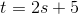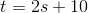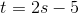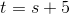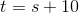Explanation:

Tommy's current age is represented by t, and Sara's is represented by s. In five years, both Tommy's and Sara's ages will be increased by five. Thus, in five years, we can represent Tommy's age as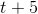and Sara's as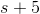.

The problem tells us that Tommy's age in five years will be twice as great as Sara's in five years. Thus, we can write an algebraic expression to represent the problem as follows: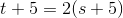In order to solve for t, first simplify the right side by distributing the 2.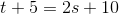Then subtract 5 from both sides.The answer is.

### Example Question #12 : Algebra

10 gallons of paint will cover 75 ft2. How many gallons of paint will be required to paint the area of a rectangular wall that has a height of 8 ft and a length of 24 ft?

17 gallons

14.2 gallons

22.8 gallons

25.6 gallons

31.4 gallons

25.6 gallons

Explanation:

First we need the area or the rectangle. 24 * 8 = 192. So now we know that 10 gallons will cover 75 ft2 and x gallons will cover 192 ft2. We set up a simple ratio and cross multiply to find that 75x = 1920.

x = 25.6

### Example Question #13 : How To Find The Solution To An Equation

What number decreased by 7 is equal to 10 increased by 7?

15

17

10

24

27

24

Explanation:

The best way to solve this problem is to translate it into an equation, "decreased" meaning subtract and "increased" meaning add:

x – 7 = 10 + 7

x = 24

### Example Question #191 : Algebra

If a%b = (2b + 3a)/(6ab), what would have a greater value, 2%3 or 3%2?

They are the same

3%2

Cannot be determined

2%3